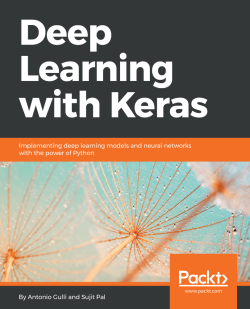# Deep Learning with Keras4.3 (22 reviews total)
By Antonio Gulli , Sujit Pal
• \$41.99 eBook
• \$51.99 Print + eBook
What do you get with a Packt Subscription?

• Instant access to this title and 7,500+ eBooks & Videos
• Constantly updated with 100+ new titles each month
• Breadth and depth in over 1,000+ technologies

This book starts by introducing you to supervised learning algorithms such as simple linear regression, the classical multilayer perceptron and more sophisticated deep convolutional networks. You will also explore image processing with recognition of hand written digit images, classification of images into different categories, and advanced objects recognition with related image annotations. An example of identification of salient points for face detection is also provided. Next you will be introduced to Recurrent Networks, which are optimized for processing sequence data such as text, audio or time series. Following that, you will learn about unsupervised learning algorithms such as Autoencoders and the very popular Generative Adversarial Networks (GAN). You will also explore non-traditional uses of neural networks as Style Transfer.

Finally, you will look at Reinforcement Learning and its application to AI game playing, another popular direction of research and application of neural networks.

Publication date:
April 2017
Publisher
Packt
Pages
318
ISBN
9781787128422

## Chapter 1. Neural Networks Foundations

Artificial neural networks (briefly, nets) represent a class of machine learning models, loosely inspired by studies about the central nervous systems of mammals. Each net is made up of several interconnected neurons, organized in layers, which exchange messages (they fire, in jargon) when certain conditions happen. Initial studies were started in the late 1950s with the introduction of the perceptron (for more information, refer to the article: The Perceptron: A Probabilistic Model for Information Storage and Organization in the Brain, by F. Rosenblatt, Psychological Review, vol. 65, pp. 386 - 408, 1958), a two-layer network used for simple operations, and further expanded in the late 1960s with the introduction of the backpropagation algorithm, used for efficient multilayer networks training (according to the articles: Backpropagation through Time: What It Does and How to Do It, by P. J. Werbos, Proceedings of the IEEE, vol. 78, pp. 1550 - 1560, 1990, and A Fast Learning Algorithm for Deep Belief Nets, by G. E. Hinton, S. Osindero, and Y. W. Teh, Neural Computing, vol. 18, pp. 1527 - 1554, 2006). Some studies argue that these techniques have roots dating further back than normally cited (for more information, refer to the article: Deep Learning in Neural Networks: An Overview, by J. Schmidhuber, vol. 61, pp. 85 - 117, 2015). Neural networks were a topic of intensive academic studies until the 1980s, when other simpler approaches became more relevant. However, there has been a resurrection of interest starting from the mid-2000s, thanks to both a breakthrough fast-learning algorithm proposed by G. Hinton (for more information, refer to the articles: The Roots of Backpropagation: From Ordered Derivatives to Neural Networks and Political Forecasting, Neural Networks, by S. Leven, vol. 9, 1996 and Learning Representations by Backpropagating Errors, by D. E. Rumelhart, G. E. Hinton, and R. J. Williams, vol. 323, 1986) and the introduction of GPUs, roughly in 2011, for massive numeric computation.

These improvements opened the route for modern deep learning, a class of neural networks characterized by a significant number of layers of neurons, which are able to learn rather sophisticated models based on progressive levels of abstraction. People called it deep with 3-5 layers a few years ago, and now it has gone up to 100-200.

This learning via progressive abstraction resembles vision models that have evolved over millions of years in the human brain. The human visual system is indeed organized into different layers. Our eyes are connected to an area of the brain called the visual cortex V1, which is located in the lower posterior part of our brain. This area is common to many mammals and has the role of discriminating basic properties and small changes in visual orientation, spatial frequencies, and colors. It has been estimated that V1 consists of about 140 million neurons, with 10 billion connections between them. V1 is then connected with other areas V2, V3, V4, V5, and V6, doing progressively more complex image processing and recognition of more sophisticated concepts, such as shapes, faces, animals, and many more. This organization in layers is the result of a huge number of attempts tuned over several 100 million years. It has been estimated that there are ~16 billion human cortical neurons, and about 10%-25% of the human cortex is devoted to vision (for more information, refer to the article: The Human Brain in Numbers: A Linearly Scaled-up Primate Brain, by S. Herculano-Houzel, vol. 3, 2009). Deep learning has taken some inspiration from this layer-based organization of the human visual system: early artificial neuron layers learn basic properties of images, while deeper layers learn more sophisticated concepts.

This book covers several major aspects of neural networks by providing working nets coded in Keras, a minimalist and efficient Python library for deep learning computations running on the top of either Google's TensorFlow (for more information, refer to https://www.tensorflow.org/) or University of Montreal's Theano (for more information, refer to http://deeplearning.net/software/theano/) backend. So, let's start.

In this chapter, we will cover the following topics:

• Perceptron
• Multilayer perceptron
• Activation functions
• Stochastic gradient descent
• Backpropagation

## Perceptron

The perceptron is a simple algorithm which, given an input vector x of m values (x1, x2, ..., xn) often called input features or simply features, outputs either 1 (yes) or 0 (no). Mathematically, we define a function: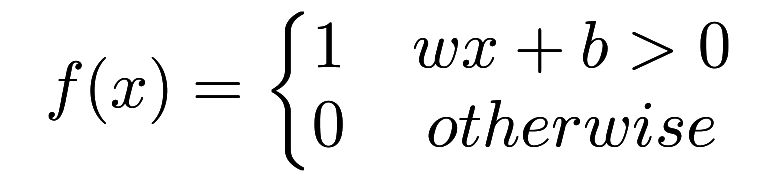Here, w is a vector of weights, wx is the dot product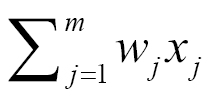, and b is a bias. If you remember elementary geometry, wx + b defines a boundary hyperplane that changes position according to the values assigned to w and b. If x lies above the straight line, then the answer is positive, otherwise it is negative. Very simple algorithm! The perception cannot express a maybe answer. It can answer yes (1) or no (0) if we understand how to define w and b, that is the training process that will be discussed in the following paragraphs.

### The first example of Keras code

The initial building block of Keras is a model, and the simplest model is called sequential. A sequential Keras model is a linear pipeline (a stack) of neural networks layers. This code fragment defines a single layer with `12` artificial neurons, and it expects `8` input variables (also known as features):

```from keras.models import Sequential
model = Sequential()

Each neuron can be initialized with specific weights. Keras provides a few choices, the most common of which are listed as follows:

• `random_uniform`: Weights are initialized to uniformly random small values in (-0.05, 0.05). In other words, any value within the given interval is equally likely to be drawn.
• `random_normal`: Weights are initialized according to a Gaussian, with a zero mean and small standard deviation of 0.05. For those of you who are not familiar with a Gaussian, think about a symmetric bell curve shape.
• `zero`: All weights are initialized to zero.

A full list is available at https://keras.io/initializations/.

## Multilayer perceptron — the first example of a network

In this chapter, we define the first example of a network with multiple linear layers. Historically, perceptron was the name given to a model having one single linear layer, and as a consequence, if it has multiple layers, you would call it multilayer perceptron (MLP). The following image represents a generic neural network with one input layer, one intermediate layer and one output layer.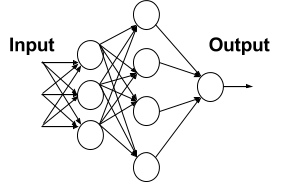In the preceding diagram, each node in the first layer receives an input and fires according to the predefined local decision boundaries. Then the output of the first layer is passed to the second layer, the results of which are passed to the final output layer consisting of one single neuron. It is interesting to note that this layered organization vaguely resembles the patterns of human vision we discussed earlier.

### Note

The net is dense, meaning that each neuron in a layer is connected to all neurons located in the previous layer and to all the neurons in the following layer.

### Problems in training the perceptron and a solution

Let's consider a single neuron; what are the best choices for the weight w and the bias b? Ideally, we would like to provide a set of training examples and let the computer adjust the weight and the bias in such a way that the errors produced in the output are minimized. In order to make this a bit more concrete, let's suppose we have a set of images of cats and another separate set of images not containing cats. For the sake of simplicity, assume that each neuron looks at a single input pixel value. While the computer processes these images, we would like our neuron to adjust its weights and bias so that we have fewer and fewer images wrongly recognized as non-cats. This approach seems very intuitive, but it requires that a small change in weights (and/or bias) causes only a small change in outputs.

If we have a big output jump, we cannot progressively learn (rather than trying things in all possible directions—a process known as exhaustive search—without knowing if we are improving). After all, kids learn little by little. Unfortunately, the perceptron does not show this little-by-little behavior. A perceptron is either 0 or 1 and that is a big jump and it will not help it to learn, as shown in the following graph: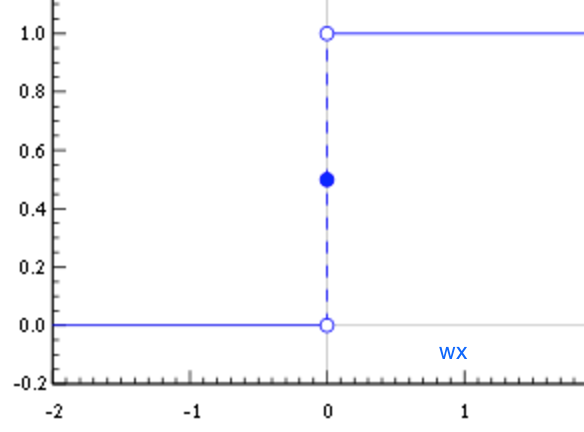We need something different, smoother. We need a function that progressively changes from 0 to 1 with no discontinuity. Mathematically, this means that we need a continuous function that allows us to compute the derivative.

### Activation function — sigmoid

The sigmoid function is defined as follows: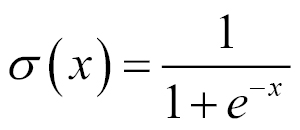As represented in the following graph, it has small output changes in (0, 1) when the input varies in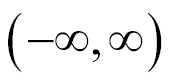. Mathematically, the function is continuous. A typical sigmoid function is represented in the following graph: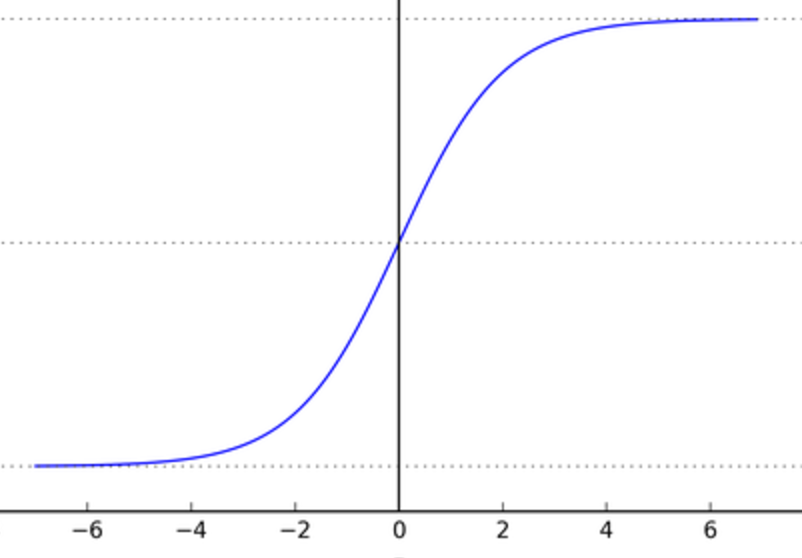A neuron can use the sigmoid for computing the nonlinear function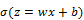. Note that, if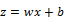is very large and positive, then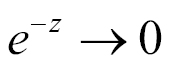, so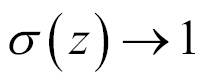, while if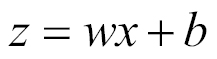is very large and negative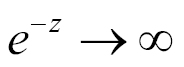so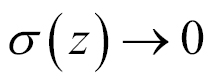. In other words, a neuron with sigmoid activation has a behavior similar to the perceptron, but the changes are gradual and output values, such as 0.5539 or 0.123191, are perfectly legitimate. In this sense, a sigmoid neuron can answer maybe.

### Activation function — ReLU

The sigmoid is not the only kind of smooth activation function used for neural networks. Recently, a very simple function called rectified linear unit (ReLU) became very popular because it generates very good experimental results. A ReLU is simply defined as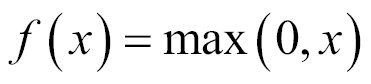, and the nonlinear function is represented in the following graph. As you can see in the following graph, the function is zero for negative values, and it grows linearly for positive values: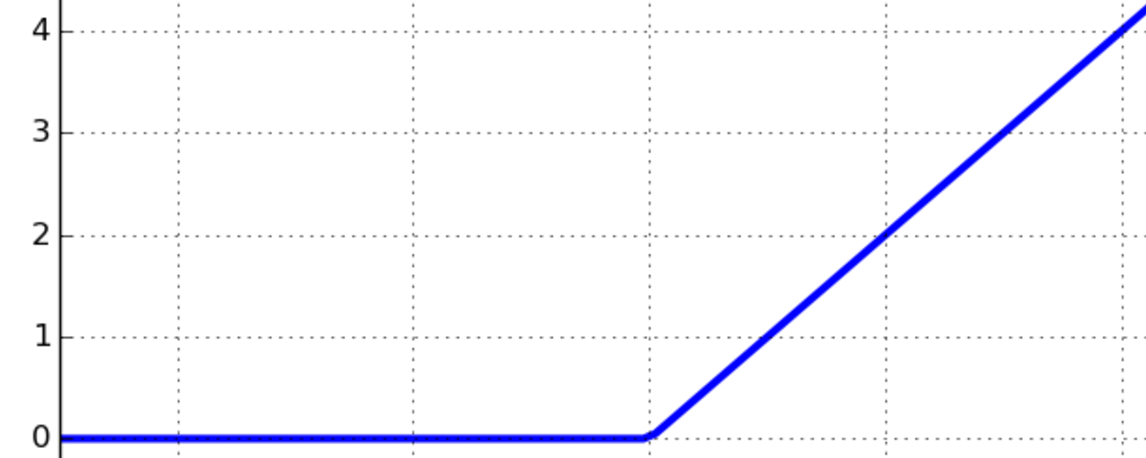### Activation functions

Sigmoid and ReLU are generally called activation functions in neural network jargon. In the Testing different optimizers in Keras section, we will see that those gradual changes, typical of sigmoid and ReLU functions, are the basic building blocks to developing a learning algorithm which adapts little by little, by progressively reducing the mistakes made by our nets. An example of using the activation function σ with the (x1, x2, ..., xm) input vector, (w1, w2, ..., wm) weight vector, b bias, and Σ summation is given in the following diagram:Keras supports a number of activation functions, and a full list is available at https://keras.io/activations/.

## A real example — recognizing handwritten digits

In this section, we will build a network that can recognize handwritten numbers. For achieving this goal, we use MNIST (for more information, refer to http://yann.lecun.com/exdb/mnist/), a database of handwritten digits made up of a training set of 60,000 examples and a test set of 10,000 examples. The training examples are annotated by humans with the correct answer. For instance, if the handwritten digit is the number three, then three is simply the label associated with that example.

In machine learning, when a dataset with correct answers is available, we say that we can perform a form of supervised learning. In this case, we can use training examples for tuning up our net. Testing examples also have the correct answer associated with each digit. In this case, however, the idea is to pretend that the label is unknown, let the network do the prediction, and then later on, reconsider the label to evaluate how well our neural network has learned to recognize digits. So, not unsurprisingly, testing examples are just used to test our net.

Each MNIST image is in gray scale, and it consists of 28 x 28 pixels. A subset of these numbers is represented in the following diagram: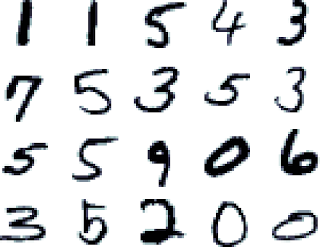### One-hot encoding — OHE

In many applications, it is convenient to transform categorical (non-numerical) features into numerical variables. For instance, the categorical feature digit with the value d in [0-9] can be encoded into a binary vector with 10 positions, which always has 0 value, except the d-th position where a 1 is present. This type of representation is called one-hot encoding (OHE) and is very common in data mining when the learning algorithm is specialized for dealing with numerical functions.

### Defining a simple neural net in Keras

Here, we use Keras to define a network that recognizes MNIST handwritten digits. We start with a very simple neural network and then progressively improve it.

Keras provides suitable libraries to load the dataset and split it into training sets `X_train`, used for fine-tuning our net, and tests set `X_test`, used for assessing the performance. Data is converted into `float32` for supporting GPU computation and normalized to [0, 1]. In addition, we load the true labels into `Y_train` and `Y_test` respectively and perform a one-hot encoding on them. Let's see the code:

```from __future__ import print_function
import numpy as np
from keras.datasets import mnist
from keras.models import Sequential
from keras.layers.core import Dense, Activation
from keras.optimizers import SGD
from keras.utils import np_utils
np.random.seed(1671) # for reproducibility

# network and training
NB_EPOCH = 200
BATCH_SIZE = 128
VERBOSE = 1
NB_CLASSES = 10 # number of outputs = number of digits
OPTIMIZER = SGD() # SGD optimizer, explained later in this chapter
N_HIDDEN = 128
VALIDATION_SPLIT=0.2 # how much TRAIN is reserved for VALIDATION

# data: shuffled and split between train and test sets
#
(X_train, y_train), (X_test, y_test) = mnist.load_data()
#X_train is 60000 rows of 28x28 values --> reshaped in 60000 x 784
RESHAPED = 784
#
X_train = X_train.reshape(60000, RESHAPED)
X_test = X_test.reshape(10000, RESHAPED)
X_train = X_train.astype('float32')
X_test = X_test.astype('float32')
# normalize
#
X_train /= 255
X_test /= 255
print(X_train.shape, 'train samples')
print(X_test.shape, 'test samples')
# convert class vectors to binary class matrices
Y_train = np_utils.to_categorical(y_train, NB_CLASSES)
Y_test = np_utils.to_categorical(y_test, NB_CLASSES)```

The input layer has a neuron associated with each pixel in the image for a total of 28 x 28 = 784 neurons, one for each pixel in the MNIST images.

Typically, the values associated with each pixel are normalized in the range [0, 1] (which means that the intensity of each pixel is divided by 255, the maximum intensity value). The output is 10 classes, one for each digit.

The final layer is a single neuron with activation function softmax, which is a generalization of the sigmoid function. Softmax squashes a k-dimensional vector of arbitrary real values into a k-dimensional vector of real values in the range (0, 1). In our case, it aggregates 10 answers provided by the previous layer with 10 neurons:

```# 10 outputs
# final stage is softmax
model = Sequential()
model.summary()```

Once we define the model, we have to compile it so that it can be executed by the Keras backend (either Theano or TensorFlow). There are a few choices to be made during compilation:

• We need to select the optimizer that is the specific algorithm used to update weights while we train our model
• We need to select the objective function that is used by the optimizer to navigate the space of weights (frequently, objective functions are called loss function, and the process of optimization is defined as a process of loss minimization)
• We need to evaluate the trained model

Some common choices for the objective function (a complete list of Keras objective functions is at https://keras.io/objectives/) are as follows:

• MSE: This is the mean squared error between the predictions and the true values. Mathematically, if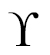is a vector of n predictions, and Y is the vector of n observed values, then they satisfy the following equation: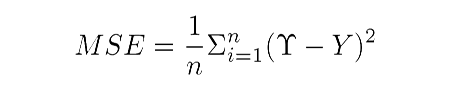### Note

These objective functions average all the mistakes made for each prediction, and if the prediction is far from the true value, then this distance is made more evident by the squaring operation.

• Binary cross-entropy: This is the binary logarithmic loss. Suppose that our model predicts p while the target is t, then the binary cross-entropy is defined as follows: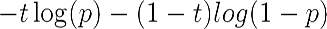### Note

This objective function is suitable for binary labels prediction.

• Categorical cross-entropy: This is the multiclass logarithmic loss. If the target is ti,j and the prediction is pi,j, then the categorical cross-entropy is this: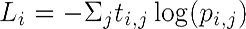### Note

This objective function is suitable for multiclass labels predictions. It is also the default choice in association with softmax activation.

Some common choices for metrics (a complete list of Keras metrics is at https://keras.io/metrics/) are as follows:

• Accuracy: This is the proportion of correct predictions with respect to the targets
• Precision: This denotes how many selected items are relevant for a multilabel classification
• Recall: This denotes how many selected items are relevant for a multilabel classification

Metrics are similar to objective functions, with the only difference that they are not used for training a model but only for evaluating a model. Compiling a model in Keras is easy:

`model.compile(loss='categorical_crossentropy', optimizer=OPTIMIZER, metrics=['accuracy'])`

Once the model is compiled, it can be then trained with the `fit()` function, which specifies a few parameters:

• `epochs`: This is the number of times the model is exposed to the training set. At each iteration, the optimizer tries to adjust the weights so that the objective function is minimized.
• `batch_size`: This is the number of training instances observed before the optimizer performs a weight update.

Training a model in Keras is very simple. Suppose we want to iterate for `NB_EPOCH` steps:

```history = model.fit(X_train, Y_train,
batch_size=BATCH_SIZE, epochs=NB_EPOCH,
verbose=VERBOSE, validation_split=VALIDATION_SPLIT)```

### Note

We reserved part of the training set for validation. The key idea is that we reserve a part of the training data for measuring the performance on the validation while training. This is a good practice to follow for any machine learning task, which we will adopt in all our examples.

Once the model is trained, we can evaluate it on the test set that contains new unseen examples. In this way, we can get the minimal value reached by the objective function and best value reached by the evaluation metric.

Note that the training set and the test set are, of course, rigorously separated. There is no point in evaluating a model on an example that has already been used for training. Learning is essentially a process intended to generalize unseen observations and not to memorize what is already known:

```score = model.evaluate(X_test, Y_test, verbose=VERBOSE)
print("Test score:", score)
print('Test accuracy:', score)```

So, congratulations, you have just defined your first neural network in Keras. A few lines of code, and your computer is able to recognize handwritten numbers. Let's run the code and see what the performance is.

### Running a simple Keras net and establishing a baseline

So let's see what will happen when we run the code in the following screenshot: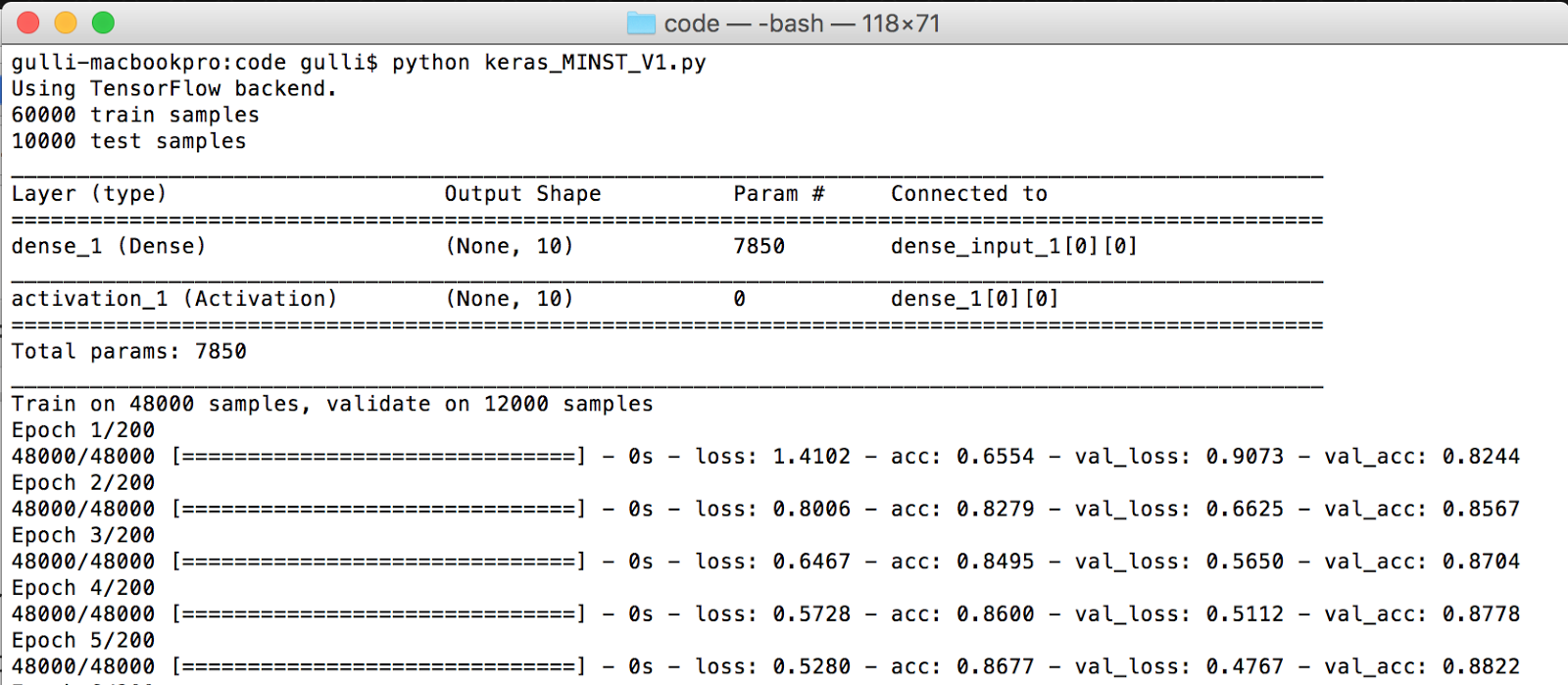First, the net architecture is dumped, and we can see the different types of layers used, their output shape, how many parameters they need to optimize, and how they are connected. Then, the network is trained on 48,000 samples, and 12,000 are reserved for validation. Once the neural model is built, it is then tested on 10,000 samples. As you can see, Keras is internally using TensorFlow as a backend system for computation. For now, we don't go into the internals on how the training happens, but we can notice that the program runs for 200 iterations, and each time, the accuracy improves. When the training ends, we test our model on the test set and achieve about 92.36% accuracy on training, 92.27% on validation, and 92.22% on the test.

This means that a bit less than one handwritten character out of ten is not correctly recognized. We can certainly do better than that. In the following screenshot, we can see the test accuracy: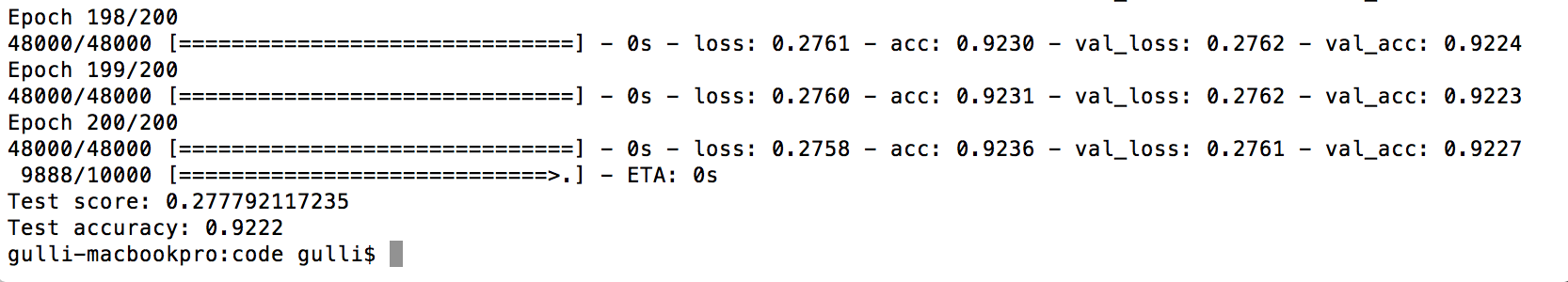### Improving the simple net in Keras with hidden layers

We have a baseline accuracy of 92.36% on training, 92.27% on validation, and 92.22% on the test. This is a good starting point, but we can certainly improve it. Let's see how.

A first improvement is to add additional layers to our network. So, after the input layer, we have a first dense layer with the `N_HIDDEN` neurons and an activation function `relu`. This additional layer is considered hidden because it is not directly connected to either the input or the output. After the first hidden layer, we have a second hidden layer, again with the `N_HIDDEN` neurons, followed by an output layer with 10 neurons, each of which will fire when the relative digit is recognized. The following code defines this new network:

```from __future__ import print_function
import numpy as np
from keras.datasets import mnist
from keras.models import Sequential
from keras.layers.core import Dense, Activation
from keras.optimizers import SGD
from keras.utils import np_utils
np.random.seed(1671) # for reproducibility
# network and training
NB_EPOCH = 20
BATCH_SIZE = 128
VERBOSE = 1
NB_CLASSES = 10 # number of outputs = number of digits
OPTIMIZER = SGD() # optimizer, explained later in this chapter
N_HIDDEN = 128
VALIDATION_SPLIT=0.2 # how much TRAIN is reserved for VALIDATION
# data: shuffled and split between train and test sets
(X_train, y_train), (X_test, y_test) = mnist.load_data()
#X_train is 60000 rows of 28x28 values --> reshaped in 60000 x 784
RESHAPED = 784
#
X_train = X_train.reshape(60000, RESHAPED)
X_test = X_test.reshape(10000, RESHAPED)
X_train = X_train.astype('float32')
X_test = X_test.astype('float32')
# normalize
X_train /= 255
X_test /= 255
print(X_train.shape, 'train samples')
print(X_test.shape, 'test samples')
# convert class vectors to binary class matrices
Y_train = np_utils.to_categorical(y_train, NB_CLASSES)
Y_test = np_utils.to_categorical(y_test, NB_CLASSES)
# M_HIDDEN hidden layers
# 10 outputs
# final stage is softmax
model = Sequential()
model.summary()
model.compile(loss='categorical_crossentropy',
optimizer=OPTIMIZER,
metrics=['accuracy'])
history = model.fit(X_train, Y_train,
batch_size=BATCH_SIZE, epochs=NB_EPOCH,
verbose=VERBOSE, validation_split=VALIDATION_SPLIT)
score = model.evaluate(X_test, Y_test, verbose=VERBOSE)
print("Test score:", score)
print('Test accuracy:', score)```

Let's run the code and see which result we get with this multilayer network. Not bad. By adding two hidden layers, we reached 94.50% on the training set, 94.63% on validation, and 94.41% on the test. This means that we gained an additional 2.2% accuracy on the test with respect to the previous network. However, we dramatically reduced the number of iterations from 200 to 20. That's good, but we want more.

If you want, you can play by yourself and see what happens if you add only one hidden layer instead of two, or if you add more than two layers. I leave this experiment as an exercise. The following screenshot shows the output of the preceding example: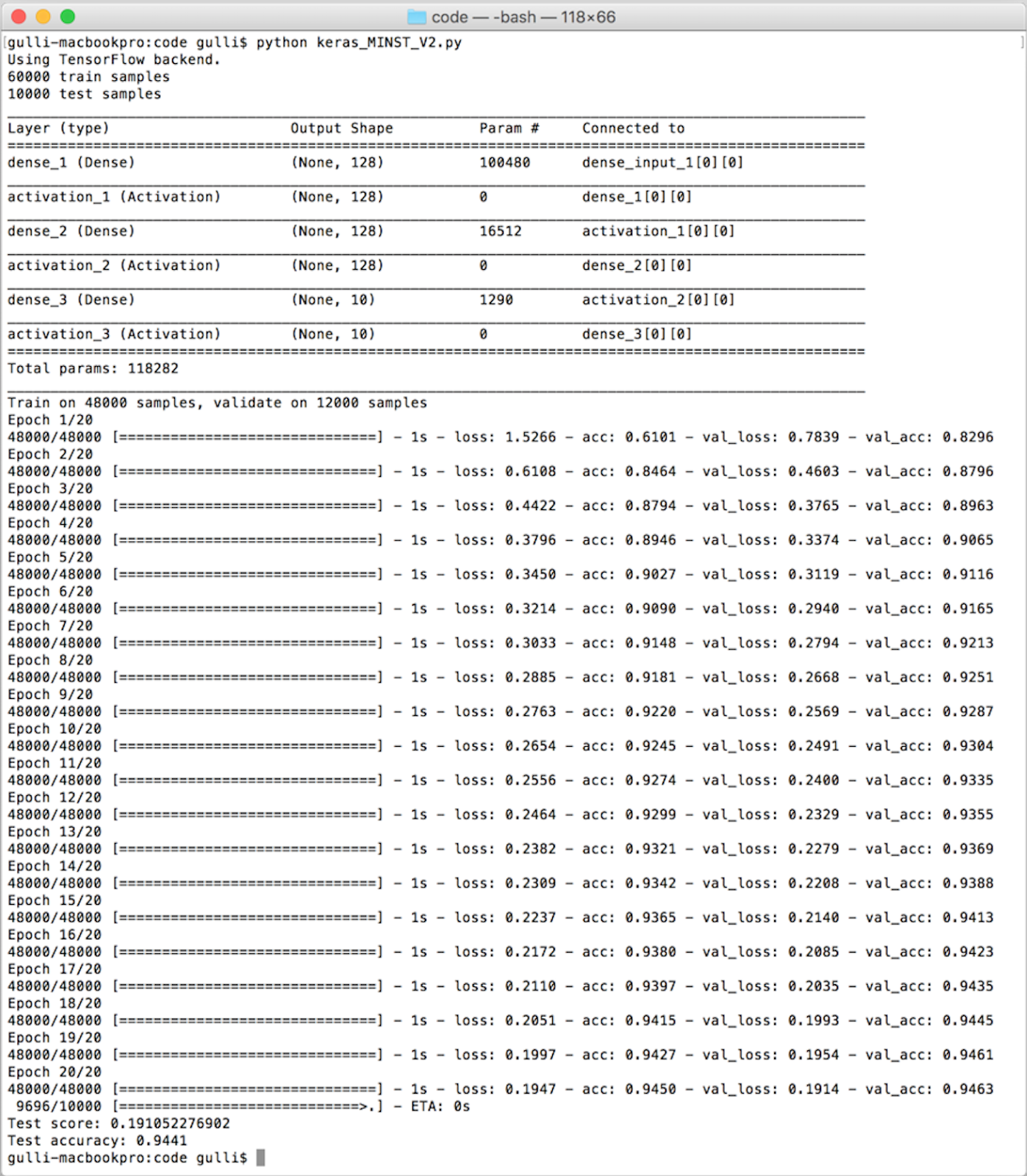### Further improving the simple net in Keras with dropout

Now our baseline is 94.50% on the training set, 94.63% on validation, and 94.41% on the test. A second improvement is very simple. We decide to randomly drop with the dropout probability some of the values propagated inside our internal dense network of hidden layers. In machine learning, this is a well-known form of regularization. Surprisingly enough, this idea of randomly dropping a few values can improve our performance:

```from __future__ import print_function
import numpy as np
from keras.datasets import mnist
from keras.models import Sequential
from keras.layers.core import Dense, Dropout, Activation
from keras.optimizers import SGD
from keras.utils import np_utils
np.random.seed(1671) # for reproducibility
# network and training
NB_EPOCH = 250
BATCH_SIZE = 128
VERBOSE = 1
NB_CLASSES = 10 # number of outputs = number of digits
OPTIMIZER = SGD() # optimizer, explained later in this chapter
N_HIDDEN = 128
VALIDATION_SPLIT=0.2 # how much TRAIN is reserved for VALIDATION
DROPOUT = 0.3
# data: shuffled and split between train and test sets
(X_train, y_train), (X_test, y_test) = mnist.load_data()
#X_train is 60000 rows of 28x28 values --> reshaped in 60000 x 784
RESHAPED = 784
#
X_train = X_train.reshape(60000, RESHAPED)
X_test = X_test.reshape(10000, RESHAPED)
X_train = X_train.astype('float32')
X_test = X_test.astype('float32')
# normalize
X_train /= 255
X_test /= 255
# convert class vectors to binary class matrices
Y_train = np_utils.to_categorical(y_train, NB_CLASSES)
Y_test = np_utils.to_categorical(y_test, NB_CLASSES)
# M_HIDDEN hidden layers 10 outputs
model = Sequential()
model.summary()
model.compile(loss='categorical_crossentropy',
optimizer=OPTIMIZER,
metrics=['accuracy'])
history = model.fit(X_train, Y_train,
batch_size=BATCH_SIZE, epochs=NB_EPOCH,
verbose=VERBOSE, validation_split=VALIDATION_SPLIT)
score = model.evaluate(X_test, Y_test, verbose=VERBOSE)
print("Test score:", score)
print('Test accuracy:', score)```

Let's run the code for 20 iterations as previously done, and we will see that this net achieves an accuracy of 91.54% on the training, 94.48% on validation, and 94.25% on the test: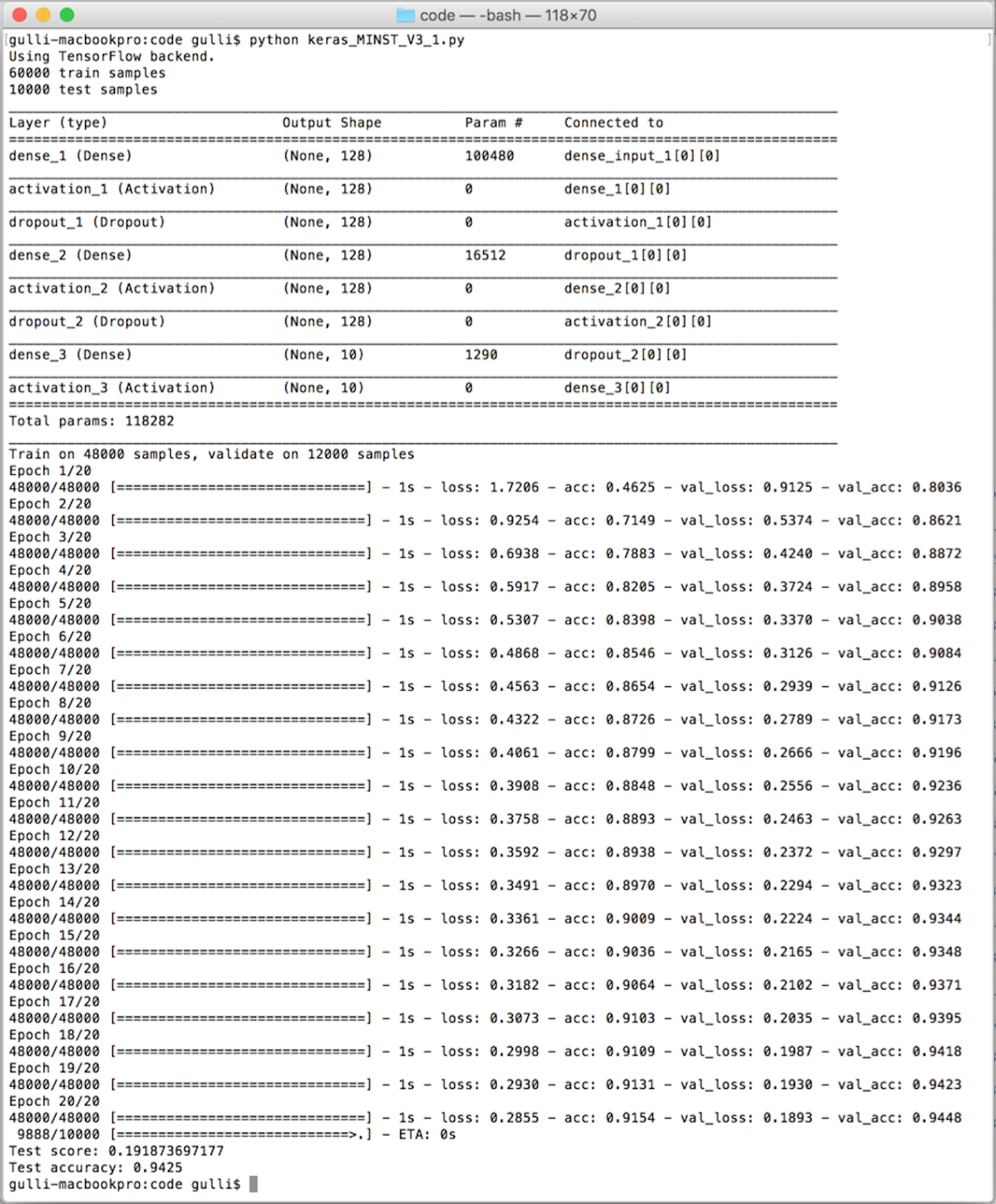Note that training accuracy should still be above the test accuracy, otherwise we are not training long enough. So let's try to increase significantly the number of epochs up to 250, and we get 98.1% accuracy on training, 97.73% on validation, and 97.7% on the test: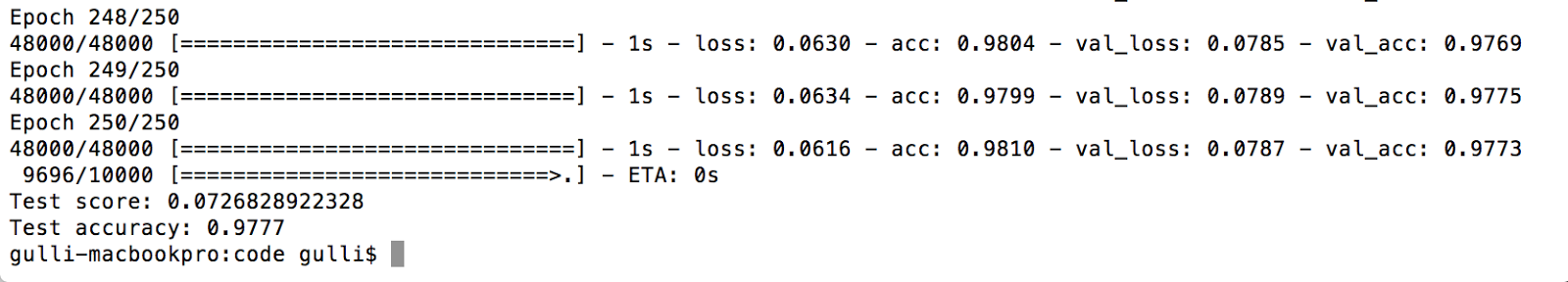It is useful to observe how accuracy increases on training and test sets when the number of epochs increases. As you can see in the following graph, these two curves touch at about 250 epochs, and therefore, there is no need to train further after that point: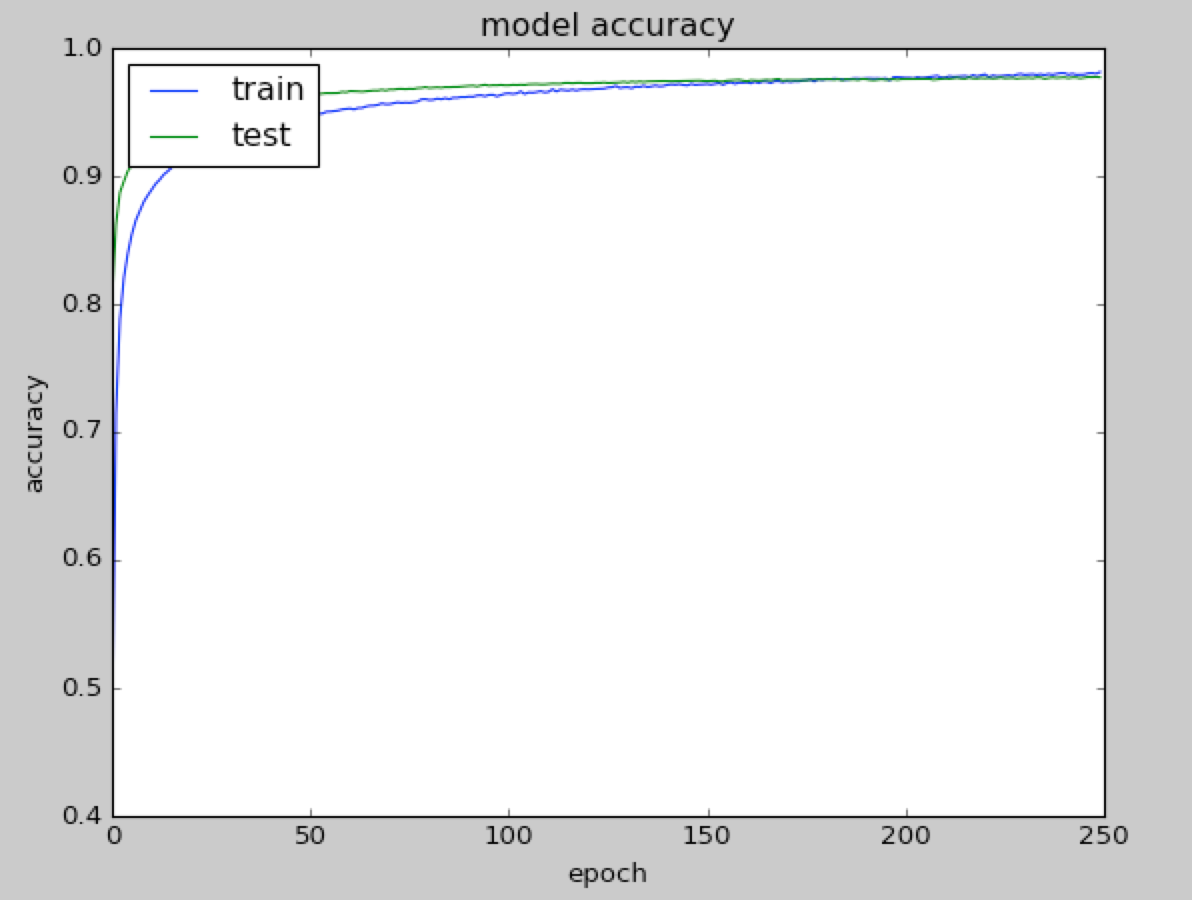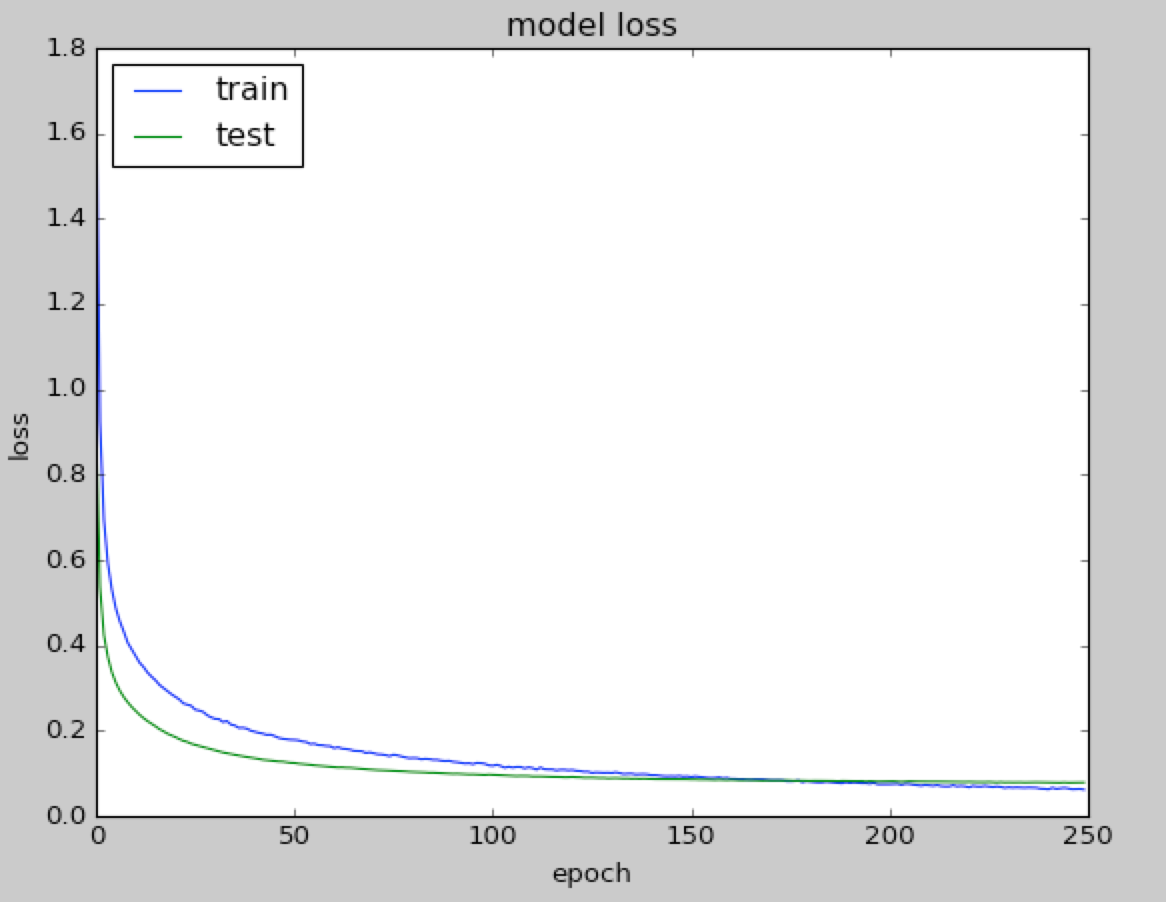Note that it has been frequently observed that networks with random dropout in internal hidden layers can generalize better on unseen examples contained in test sets. Intuitively, one can think of this as each neuron becoming more capable because it knows it cannot depend on its neighbors. During testing, there is no dropout, so we are now using all our highly tuned neurons. In short, it is generally a good approach to test how a net performs when some dropout function is adopted.

### Testing different optimizers in Keras

We have defined and used a network; it is useful to start giving an intuition about how networks are trained. Let's focus on one popular training technique known as gradient descent (GD). Imagine a generic cost function C(w) in one single variable w like in the following graph: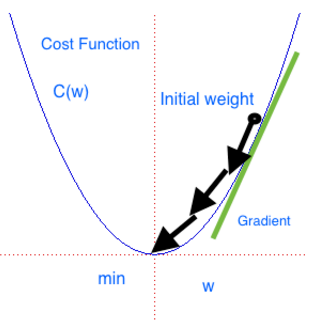The gradient descent can be seen as a hiker who aims at climbing down a mountain into a valley. The mountain represents the function C, while the valley represents the minimum Cmin. The hiker has a starting point w0. The hiker moves little by little. At each step r, the gradient is the direction of maximum increase. Mathematically, this direction is the value of the partial derivative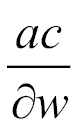evaluated at point wr reached at step r. Therefore by taking the opposite direction,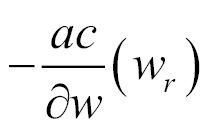, the hiker can move towards the valley. At each step, the hiker can decide what the leg length is before the next step. This is the learning rate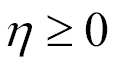in gradient descent jargon. Note that if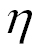is too small, then the hiker will move slowly. However, ifis too high, then the hiker will possibly miss the valley.

Now you should remember that a sigmoid is a continuous function, and it is possible to compute the derivative. It can be proven that the sigmoid is shown as follows: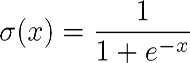It has the following derivative: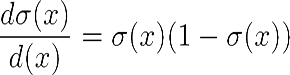ReLU is not differentiable in 0. We can, however, extend the first derivative in 0 to a function over the whole domain by choosing it to be either 0 or 1. The point-wise derivative of ReLU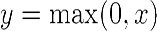is as follows: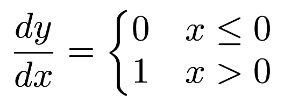Once we have the derivative, it is possible to optimize the nets with a gradient descent technique. Keras uses its backend (either TensorFlow or Theano) for computing the derivative on our behalf so we don't need to worry about implementing or computing it. We just choose the activation function, and Keras computes its derivative on our behalf.

A neural network is essentially a composition of multiple functions with thousands, and sometimes millions, of parameters. Each network layer computes a function whose error should be minimized in order to improve the accuracy observed during the learning phase. When we discuss backpropagation, we will discover that the minimization game is a bit more complex than our toy example. However, it is still based on the same intuition of descending a valley.

Keras implements a fast variant of gradient descent known as stochastic gradient descent (SGD) and two more advanced optimization techniques known as RMSprop and Adam. RMSprop and Adam include the concept of momentum (a velocity component) in addition to the acceleration component that SGD has. This allows faster convergence at the cost of more computation. A full list of Keras-supported optimizers is at https://keras.io/optimizers/. SGD was our default choice so far. So now let's try the other two. It is very simple, we just need to change few lines:

```from keras.optimizers import RMSprop, Adam
...
OPTIMIZER = RMSprop() # optimizer,```

That's it. Let's test it as shown in the following screenshot: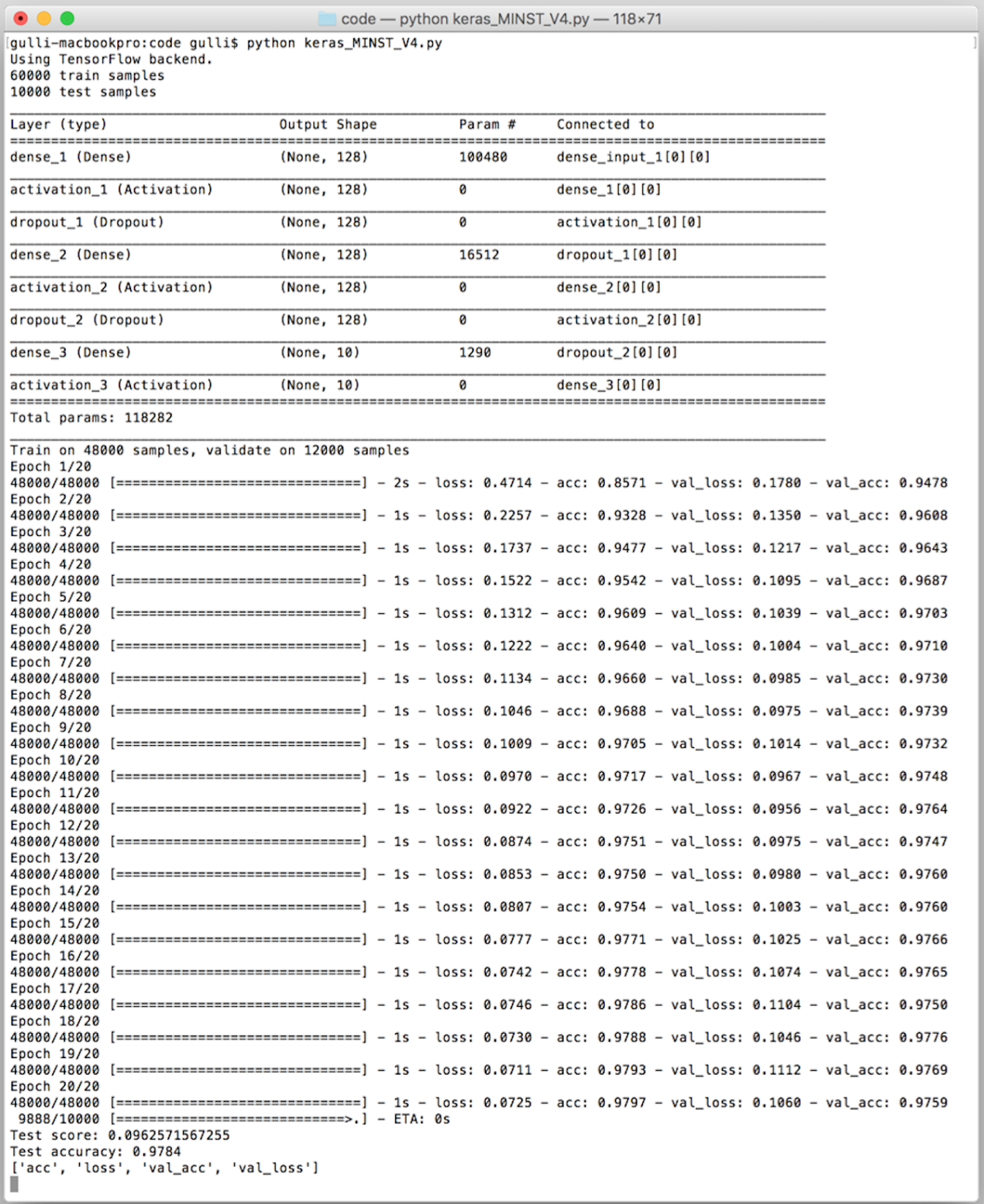As you can see in the preceding screenshot, RMSprop is faster than SDG since we are able to achieve an accuracy of 97.97% on training, 97.59% on validation, and 97.84% on the test improving SDG with only 20 iterations. For the sake of completeness, let's see how the accuracy and loss change with the number of epochs, as shown in the following graphs: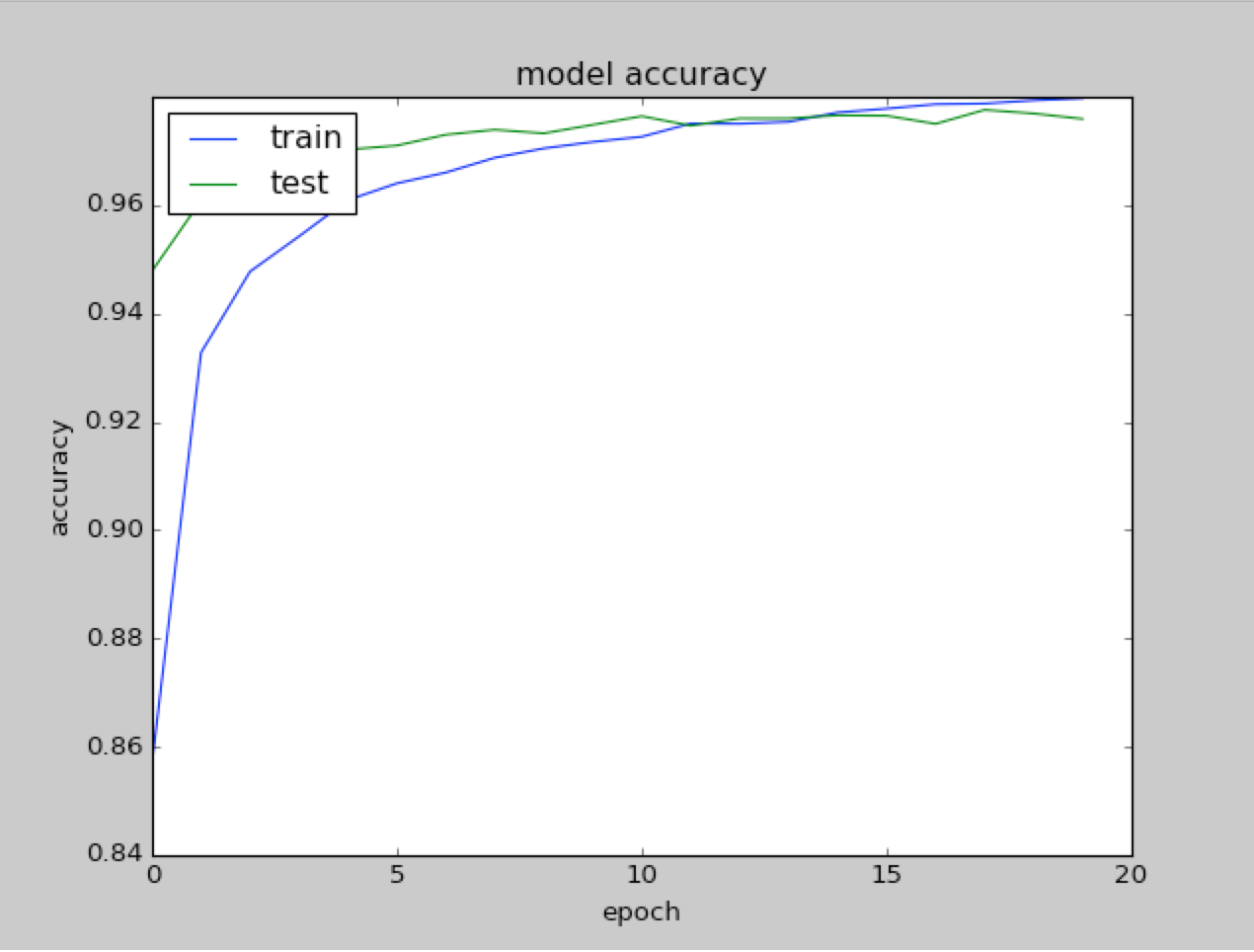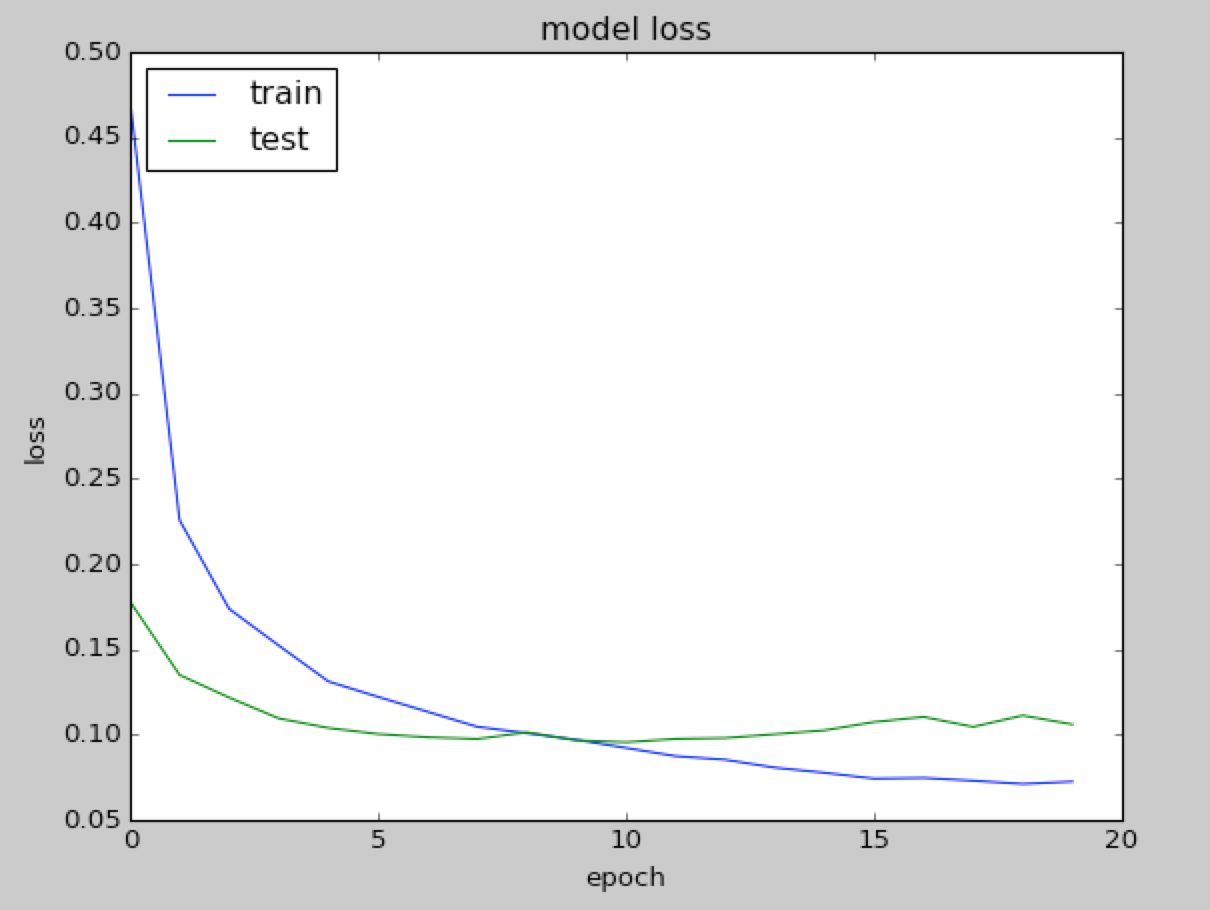OK, let's try the other optimizer, `Adam()`. It is pretty simple, as follows:

`OPTIMIZER = Adam() # optimizer`

As we have seen, Adam is slightly better. With Adam, we achieve 98.28% accuracy on training, 98.03% on validation, and 97.93% on the test with 20 iterations, as shown in the following graphs: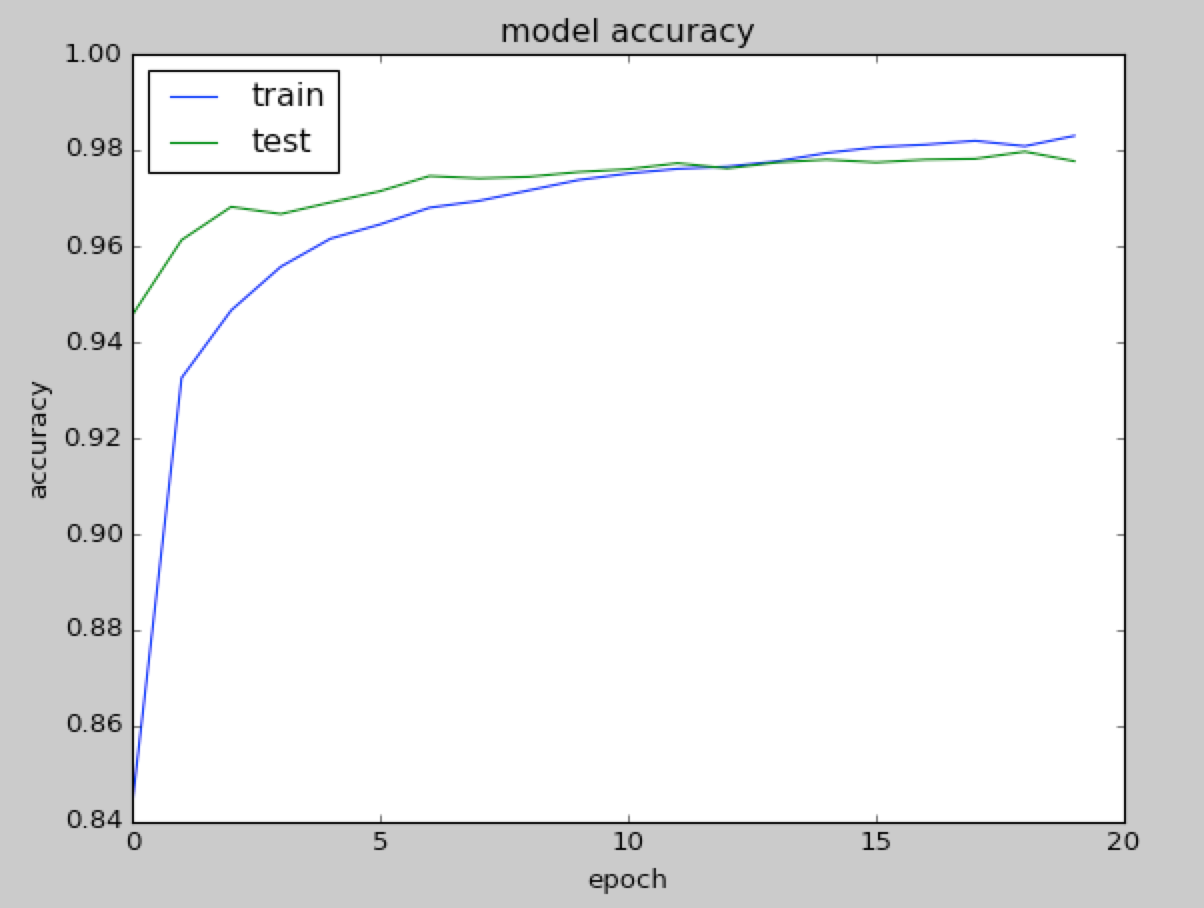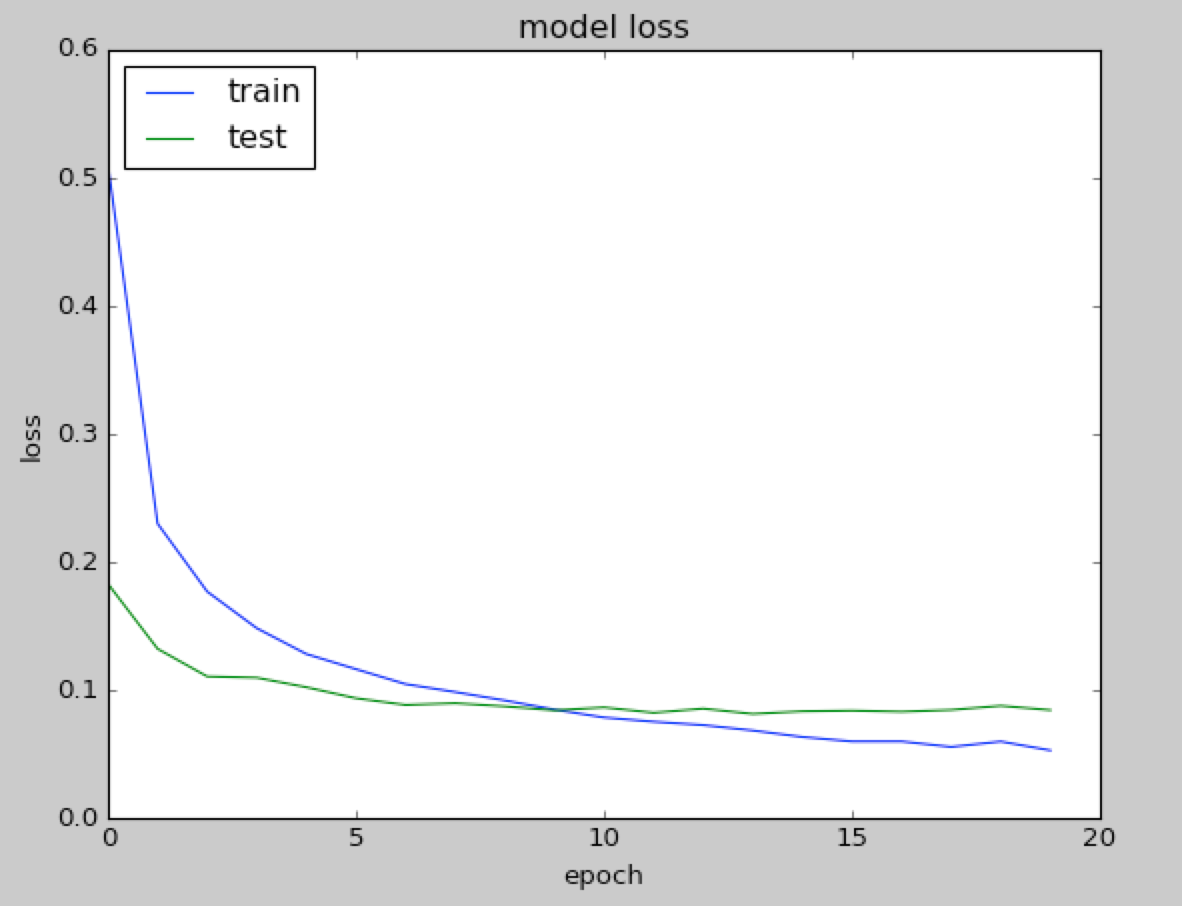This is our fifth variant, and remember that our initial baseline was at 92.36%.

So far, we made progressive improvements; however, the gains are now more and more difficult. Note that we are optimizing with a dropout of 30%. For the sake of completeness, it could be useful to report the accuracy on the test only for other dropout values with `Adam()` chosen as optimizer, as shown in the following graph: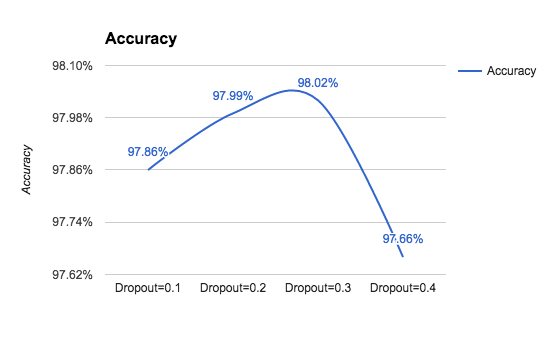### Increasing the number of epochs

Let's make another attempt and increase the number of epochs used for training from 20 to 200. Unfortunately, this choice increases our computation time by 10, but it gives us no gain. The experiment is unsuccessful, but we have learned that if we spend more time learning, we will not necessarily improve. Learning is more about adopting smart techniques and not necessarily about the time spent in computations. Let's keep track of our sixth variant in the following graph: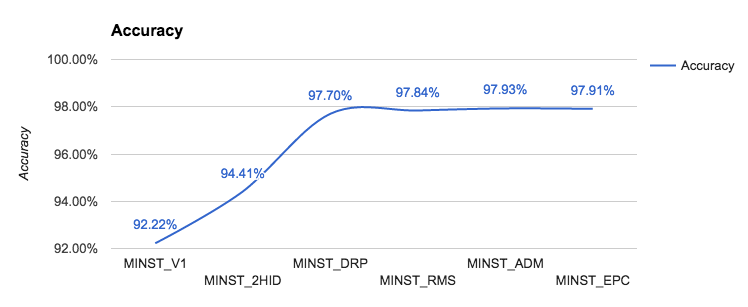### Controlling the optimizer learning rate

There is another attempt we can make, which is changing the learning parameter for our optimizer. As you can see in the following graph, the optimal value is somewhere close to 0.001, which is the default learning rate for the optimer. Good! Adam works well out of the box: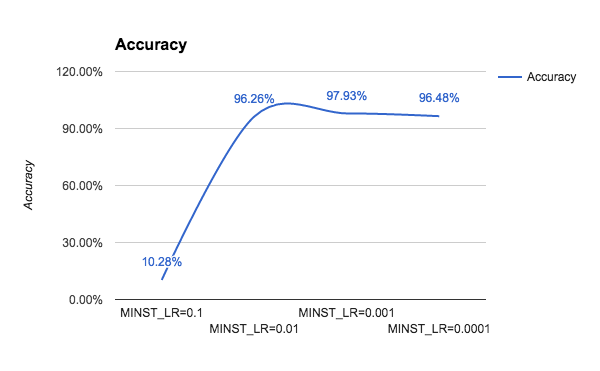### Increasing the number of internal hidden neurons

We can make yet another attempt, that is, changing the number of internal hidden neurons. We report the results of the experiments with an increasing number of hidden neurons. We can see in the following graph that by increasing the complexity of the model, the run time increases significantly because there are more and more parameters to optimize. However, the gains that we are getting by increasing the size of the network decrease more and more as the network grows: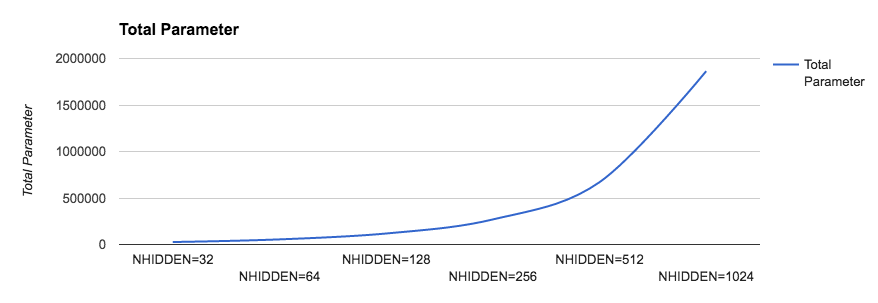In the following graph, we show the time needed for each iteration as the number of hidden neurons grow: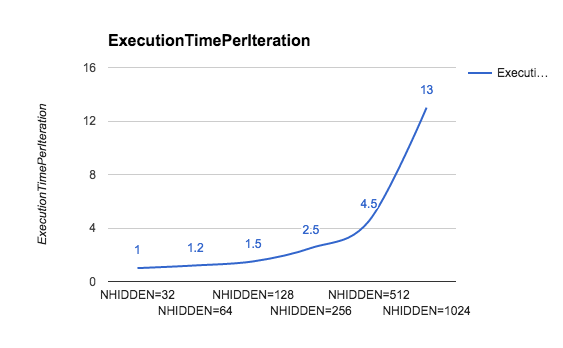The following graph shows the accuracy as the number of hidden neurons grow: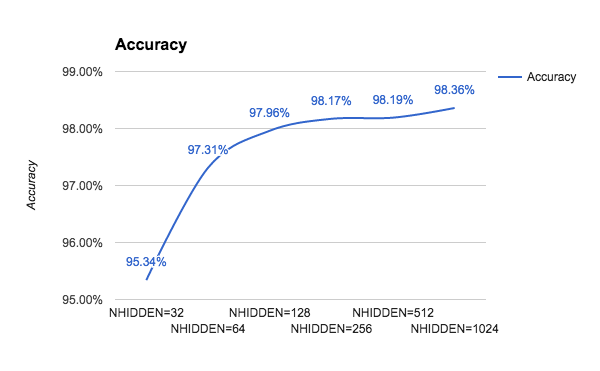### Increasing the size of batch computation

Gradient descent tries to minimize the cost function on all the examples provided in the training sets and, at the same time, for all the features provided in the input. Stochastic gradient descent is a much less expensive variant, which considers only `BATCH_SIZE` examples. So, let's see what the behavior is by changing this parameter. As you can see, the optimal accuracy value is reached for `BATCH_SIZE=128`: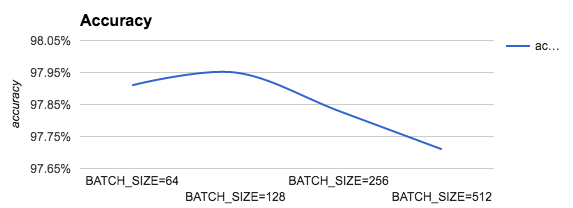### Summarizing the experiments run for recognizing handwritten charts

So, let's summarize: with five different variants, we were able to improve our performance from 92.36% to 97.93%. First, we defined a simple layer network in Keras. Then, we improved the performance by adding some hidden layers. After that, we improved the performance on the test set by adding a few random dropouts to our network and by experimenting with different types of optimizers. Current results are summarized in the following table:

 Model/Accuracy Training Validation Test Simple 92.36% 92.37% 92.22% Two hidden (128) 94.50% 94.63% 94.41% Dropout (30%) 98.10% 97.73% 97.7% (200 epochs) RMSprop 97.97% 97.59% 97.84% (20 epochs) Adam 98.28% 98.03% 97.93% (20 epochs)

However, the next two experiments did not provide significant improvements. Increasing the number of internal neurons creates more complex models and requires more expensive computations, but it provides only marginal gains. We get the same experience if we increase the number of training epochs. A final experiment consisted in changing the `BATCH_SIZE` for our optimizer.

### Adopting regularization for avoiding overfitting

Intuitively, a good machine learning model should achieve low error on training data. Mathematically, this is equivalent to minimizing the loss function on the training data given the machine learning model built. This is expressed by the following formula.: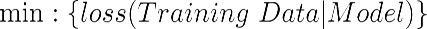However, this might not be enough. A model can become excessively complex in order to capture all the relations inherently expressed by the training data. This increase of complexity might have two negative consequences. First, a complex model might require a significant amount of time to be executed. Second, a complex model can achieve very good performance on training data—because all the inherent relations in trained data are memorized, but not so good performance on validation data—as the model is not able to generalize on fresh unseen data. Again, learning is more about generalization than memorization. The following graph represents a typical loss function decreasing on both validation and training sets. However, a certain point the loss on validation starts to increase because of overfitting: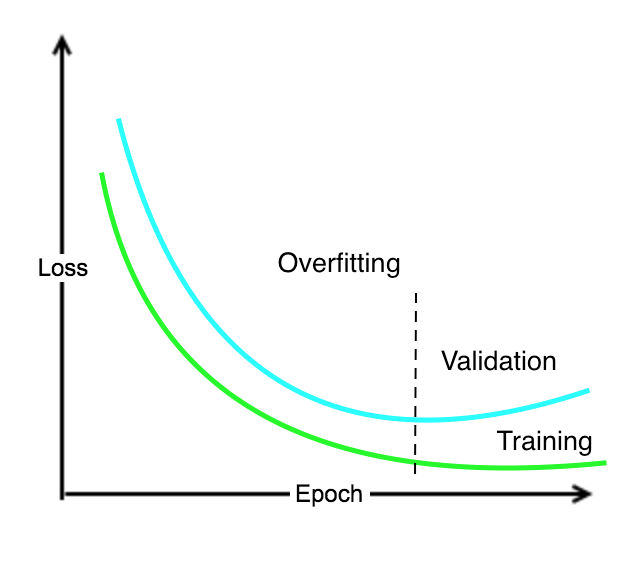As a rule of thumb, if during the training we see that the loss increases on validation, after an initial decrease, then we have a problem of model complexity that overfits training. Indeed, overfitting is the word used in machine learning for concisely describing this phenomenon.

In order to solve the overfitting problem, we need a way to capture the complexity of a model, that is, how complex a model can be. What could be the solution? Well, a model is nothing more than a vector of weights. Therefore the complexity of a model can be conveniently represented as the number of nonzero weights. In other words, if we have two models, M1 and M2, achieving pretty much the same performance in terms of loss function, then we should choose the simplest model that has the minimum number of nonzero weights. We can use a hyperparameter ⅄>=0 for controlling what the importance of having a simple model is, as in this formula: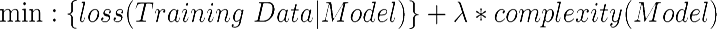There are three different types of regularizations used in machine learning:

• L1 regularization (also known as lasso): The complexity of the model is expressed as the sum of the absolute values of the weights
• L2 regularization (also known as ridge): The complexity of the model is expressed as the sum of the squares of the weights
• Elastic net regularization: The complexity of the model is captured by a combination of the two preceding techniques

Note that the same idea of regularization can be applied independently to the weights, to the model, and to the activation.

Therefore, playing with regularization can be a good way to increase the performance of a network, in particular when there is an evident situation of overfitting. This set of experiments is left as an exercise for the interested reader.

Note that Keras supports both l1, l2, and elastic net regularizations. Adding regularization is easy; for instance, here we have a `l2` regularizer for kernel (the weight W):

`from keras import regularizers model.add(Dense(64, input_dim=64, kernel_regularizer=regularizers.l2(0.01)))`

A full description of the available parameters is available at: https://keras.io/regularizers/.

### Hyperparameters tuning

The preceding experiments gave a sense of what the opportunities for fine-tuning a net are. However, what is working for this example is not necessarily working for other examples. For a given net, there are indeed multiple parameters that can be optimized (such as the number of `hidden neurons`, `BATCH_SIZE`, number of `epochs`, and many more according to the complexity of the net itself).

Hyperparameter tuning is the process of finding the optimal combination of those parameters that minimize cost functions. The key idea is that if we have n parameters, then we can imagine that they define a space with n dimensions, and the goal is to find the point in this space which corresponds to an optimal value for the cost function. One way to achieve this goal is to create a grid in this space and systematically check for each grid vertex what the value assumed by the cost function is. In other words, the parameters are divided into buckets, and different combinations of values are checked via a brute force approach.

### Predicting output

When a net is trained, it can be course be used for predictions. In Keras, this is very simple. We can use the following method:

```# calculate predictions
predictions = model.predict(X)```

For a given input, several types of output can be computed, including a method:

• `model.evaluate()`: This is used to compute the loss values
• `model.predict_classes()`: This is used to compute category outputs
• `model.predict_proba()`: This is used to compute class probabilities

## A practical overview of backpropagation

Multilayer perceptrons learn from training data through a process called backpropagation. The process can be described as a way of progressively correcting mistakes as soon as they are detected. Let's see how this works.

Remember that each neural network layer has an associated set of weights that determines the output values for a given set of inputs. In addition to that, remember that a neural network can have multiple hidden layers.

In the beginning, all the weights have some random assignment. Then the net is activated for each input in the training set: values are propagated forward from the input stage through the hidden stages to the output stage where a prediction is made (note that we have kept the following diagram simple by only representing a few values with green dotted lines, but in reality, all the values are propagated forward through the network):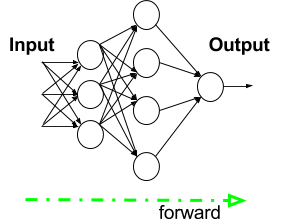Since we know the true observed value in the training set, it is possible to calculate the error made in prediction. The key intuition for backtracking is to propagate the error back and use an appropriate optimizer algorithm, such as a gradient descent, to adjust the neural network weights with the goal of reducing the error (again for the sake of simplicity, only a few error values are represented):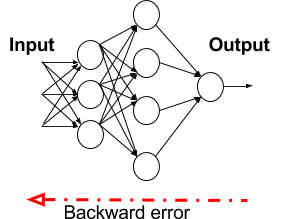The process of forward propagation from input to output and backward propagation of errors is repeated several times until the error gets below a predefined threshold. The whole process is represented in the following diagram: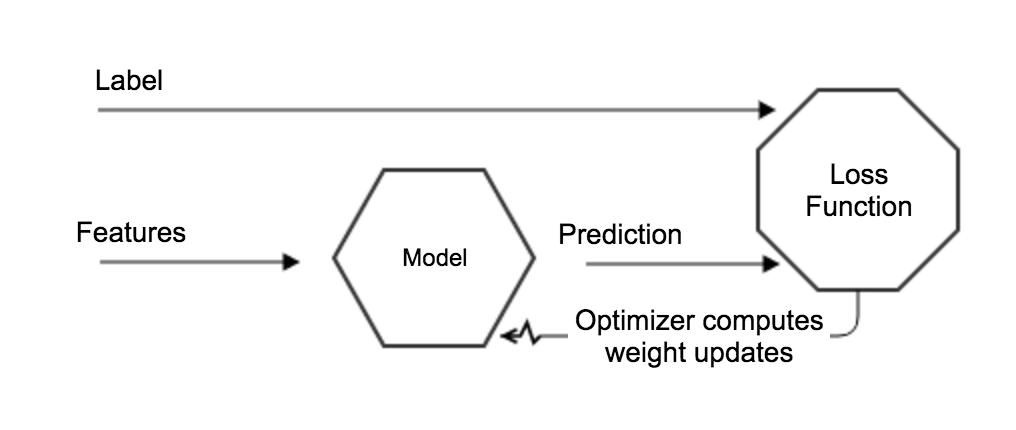The features represent the input and the labels are here used to drive the learning process. The model is updated in such a way that the loss function is progressively minimized. In a neural network, what really matters is not the output of a single neuron but the collective weights adjusted in each layer. Therefore, the network progressively adjusts its internal weights in such a way that the prediction increases the number of labels correctly forecasted. Of course, using the right set features and having a quality labeled data is fundamental to minimizing the bias during the learning process.

## Towards a deep learning approach

While playing with handwritten digit recognition, we came to the conclusion that the closer we get to the accuracy of 99%, the more difficult it is to improve. If we want to have more improvements, we definitely need a new idea. What are we missing? Think about it.

The fundamental intuition is that, so far, we lost all the information related to the local spatiality of the images. In particular, this piece of code transforms the bitmap, representing each written digit into a flat vector where the spatial locality is gone:

```#X_train is 60000 rows of 28x28 values --> reshaped in 60000 x 784
X_train = X_train.reshape(60000, 784)
X_test = X_test.reshape(10000, 784)```

However, this is not how our brain works. Remember that our vision is based on multiple cortex levels, each one recognizing more and more structured information, still preserving the locality. First we see single pixels, then from that, we recognize simple geometric forms and then more and more sophisticated elements such as objects, faces, human bodies, animals and so on.

In Chapter 3, Deep Learning with ConvNets, we will see that a particular type of deep learning network known as convolutional neural network (CNN) has been developed by taking into account both the idea of preserving the spatial locality in images (and, more generally, in any type of information) and the idea of learning via progressive levels of abstraction: with one layer, you can only learn simple patterns; with more than one layer, you can learn multiple patterns. Before discussing CNN, we need to discuss some aspects of Keras architecture and have a practical introduction to a few additional machine learning concepts. This will be the topic of the next chapters.

## Summary

In this chapter, you learned the basics of neural networks, more specifically, what a perceptron is, what a multilayer perceptron is, how to define neural networks in Keras, how to progressively improve metrics once a good baseline is established, and how to fine-tune the hyperparameter's space. In addition to that, you now also have an intuitive idea of what some useful activation functions (sigmoid and ReLU) are, and how to train a network with backpropagation algorithms based on either gradient descent, on stochastic gradient descent, or on more sophisticated approaches, such as Adam and RMSprop.

In the next chapter, we will see how to install Keras on AWS, Microsoft Azure, Google Cloud, and on your own machine. In addition to that, we will provide an overview of Keras APIs.

### About the Authors

• ##### Antonio Gulli

Antonio Gulli is a software executive and business leader with a passion for establishing and managing global technological talent, innovation, and execution. He is an expert in search engines, online services, machine learning, information retrieval, analytics, and cloud computing. So far, he has been lucky enough to gain professional experience in four different countries in Europe and has managed people in six different countries in Europe and America. Antonio served as CEO, GM, CTO, VP, director, and site lead in multiple fields ranging from publishing (Elsevier) to consumer internet (Ask.com and Tiscali) and high-tech R&D (Microsoft and Google).

Browse publications by this author
• ##### Sujit Pal

Sujit Pal is a technology research director at Elsevier Labs, working on building intelligent systems around research content and metadata. His primary interests are information retrieval, ontologies, natural language processing, machine learning, and distributed processing. He is currently working on image classification and similarity using deep learning models. Prior to this, he worked in the consumer healthcare industry, where he helped build ontology-backed semantic search, contextual advertising, and EMR data processing platforms. He writes about technology on his blog at Salmon Run.

Browse publications by this author

### Latest Reviews

(22 reviews total)